# NCERT Solutions for Class 10 Science Chapter 10 Light Reflection and Refraction

In this page we have provided NCERT Solutions for Class 10 Science Chapter 10 Light Reflection and Refraction through which you can complete your homework on time and increase concentration as well. These Chapter 10 NCERT questions and answers of Class 10 Science will help you in analyzing the problems and answering it with precision and the right concepts.

In this chapter, we will learn about variety of topics like  laws of reflection, propagation of light, plane mirror, spherical mirror, mirror formula, laws of refraction and spherical lenses which will widen your perspective of knowledge. You can figure out the latest marking scheme and prepare your answers as per the demand with the help of these Class 10 NCERT Solutions.Study Reference for Class 10 Chapter 10 Light Reflection and Refraction

In Text Questions

Page No: 168

1. Define the principal focus of a concave mirror.

Light rays that are parallel to the principal axis of a concave mirror converge at a specific point on its principal axis after reflecting from the mirror. This point is known as the principal focus of the concave mirror.

2. The radius of curvature of a spherical mirror is 20 cm. What is its focal length?

Radius of curvature, R= 20 cm
Radius of curvature of a spherical mirror = 2 × Focal length (f)R= 2f
f= R/2 = 20 / 2 = 10
Hence, the focal length of the given spherical mirror is 10 cm.

3. Name the mirror that can give an erect and enlarged image of an object.
► Concave Mirror.

4. Why do we prefer a convex mirror as a rear-view mirror in vehicles?

We prefer a convex mirror as a rear-view mirror in vehicles because it gives a wider field of view, which allows the driver to see most of the traffic behind him. Convex mirrors always form a virtual, erect, and diminished image of the objects placed in front of it.

Page No: 171

1. Find the focal length of a convex mirror whose radius of curvature is 32 cm.

Radius of curvature, R= 32 cm
Radius of curvature = 2 × Focal length (f)
R= 2ff= R/2 = 32/2 = 16
Hence, the focal length of the given convex mirror is 16 cm.

2. A concave mirror produces three times magnified (enlarged) real image of object placed at 10 cm in front of it. Where is the image located?

Magnification produced by a spherical mirror is given by the relation,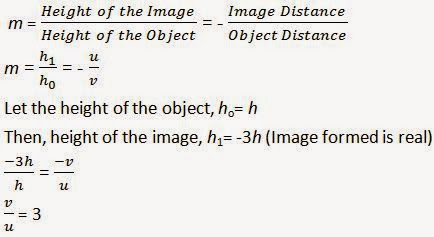Object distance, u= - 10 cm
= 3 × ( - 10) = - 30 cm
Here, the negative sign indicates that an inverted image is formed at a distance of
30 cm in front of the given concave mirror.

Page No: 176

1. A ray of light travelling in air enters obliquely into water. Does the light ray bend towards the normal or away from the normal? Why?

The ray of light bends towards the normal. When a ray of light enters from an optically rarer medium (having low refractive index) to an optically denser medium (having high refractive index), its speed slows down and it bends towards the normal. Since water is optically denser than air, a ray of light entering from air into water will bend towards the normal.

2. Light enters from air to glass having refractive index 1.50. What is the speed of light in the glass? The speed of light in vacuum is 3 x 108 ms-1.

Refractive index of a medium, nm = Speed of light in vacuum/Speed of light in the medium
Speed of light in vacuum, c = 3 × 108 ms-1
Refractive index of glass, ng = 1.50
Speed of light in the glass, v = Speed of light in vacuum/ Refractive index of glass
= c/ng
=3 × 108/1.50 = 2x 108 ms-1.

3. Find out, from Table, the medium having highest optical density. Also find the medium with lowest optical density.
 Material medium Refractive index Material medium Refractive index Air 1.0003 Canada Balsam 1.53 Ice 1.31 - - Water 1.33 Rock salt 1.54 Alcohol 1.36 - - Kerosene 1.44 Carbon disulphide 1.63 Fused quartz 1.46 Dense flint glass 1.65 Turpentine oil 1.47 Ruby 1.71 Benzene 1.50 Sapphire 1.77 Crown glass 1.52 Diamond 2.42

Highest optical density = Diamond
Lowest optical density = Air
Optical density of a medium is directly related with the refractive index of that medium. A medium which has the highest refractive index will have the highest optical density and vice-versa.

It can be observed from table 10.3 that diamond and air respectively have the highest and lowest refractive index. Therefore, diamond has the highest optical density and air has the lowest optical
density.

4. You are given kerosene, turpentine and water. In which of these does the light travel fastest? Use the information given in Table.
 Material medium Refractive index Material medium Refractive index Air 1.0003 Canada Balsam 1.53 Ice 1.31 - - Water 1.33 Rock salt 1.54 Alcohol 1.36 - - Kerosene 1.44 Carbon disulphide 1.63 Fused quartz 1.46 Dense flint glass 1.65 Turpentine oil 1.47 Ruby 1.71 Benzene 1.50 Sapphire 1.77 Crown glass 1.52 Diamond 2.42

In water light travel faster as compare to kerosene and turpentine because the refractive index of water is lower than that of kerosene and turpentine. The speed of light is inversely proportional to the refractive index.

5. The refractive index of diamond is 2.42. What is the meaning of this statement?

The refractive index of diamond is 2.42. This means that the speed of light in diamond will reduce by a factor of 2.42 as compared to its speed in air.
In other words, the speed of light in diamond is 1/2.42 times the speed of light in vacuum.

Page No: 184

1. Define 1 dioptre of power of a lens.

The SI unit of power of lens is dioptre which is denoted by the letter D. 1 dioptre is defined as the power of a lens of focal length 1 metre.

2. A convex lens forms a real and inverted image of a needle at a distance of 50 cm from it. Where is the needle placed in front of the convex lens if the image is equal to the size of the object? Also, find the power of the lens.

Since the image is real and same size. The position of image should be at 2F.

It is given that the image of the needle is formed at a distance of 50 cm from the convex lens. Hence, the needle is placed in front of the lens at a distance of 50 cm.

Object distance, u= - 50 cm
Image distance, v= 50 cm
Focal length = f
According to the lens formula,3. Find the power of a concave lens of focal length 2 m.

Focal length of concave lens, f = 2 mPower of lens, P= 1/f = 1/(-2)= -0.5D

Page No: 185

Exercise

1. Which one of the following materials cannot be used to make a lens?
(a) Water
(b) Glass
(c) Plastic
(d) Clay
► (d) Clay

2. The image formed by a concave mirror is observed to be virtual, erect and larger than the object. Where should be the position of the object?
(a) Between the principal focus and the centre of curvature
(b) At the centre of curvature
(c) Beyond the centre of curvature
(d) Between the pole of the mirror and its principal focus.
► (d) Between the pole of the mirror and its principal focus.

3. Where should an object be placed in front of a convex lens to get a real image of the size of the object?
(a) At the principal focus of the lens
(b) At twice the focal length
(c) At infinity
(d) Between the optical centre of the lens and its principal focus.
► (b) At twice the focal length

4. A spherical mirror and a thin spherical lens have each a focal length of -15 cm. The mirror and the lens are likely to be
(a) both concave
(b) both convex
(c) the mirror is concave and the lens is convex
(d) the mirror is convex, but the lens is concave
► (a) both concave

Page No: 186

5. No matter how far you stand from a mirror, your image appears erect. The mirror is likely to be
(a) plane
(b) concave
(c) convex
(d) either plane or convex
► (d) either plane or convex

6. Which of the following lenses would you prefer to use while reading small letters found in a dictionary?
(a) A convex lens of focal length 50 cm
(b) A concave lens of focal length 50 cm
(c) A convex lens of focal length 5 cm
(d) A concave lens of focal length 5 cm
► (c) A convex lens of focal length 5 cm

7. We wish to obtain an erect image of an object, using a concave mirror of focal length 15 cm. What should be the range of distance of the object from the mirror? What is the nature of the image? Is the image larger or smaller than the object? Draw a ray diagram to show the image formation in this case.

Range of the distance of the object = 0 to 15 cm from the pole of the mirror.
Nature of the image = virtual, erect and larger than the object.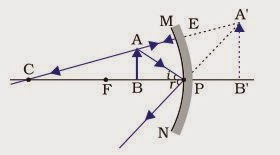8. Name the type of mirror used in the following situations.
(b) Side/rear-view mirror of a vehicle
(c) Solar furnace

(a) Concave Mirror: This is because concave mirrors can produce powerful parallel beam of light when the light source is placed at their principal focus.

(b) Convex Mirror: This is because of its largest field of view.

(c) Concave Mirror: This is because it concentrates the parallel rays of sun at principal focus.

9. One-half of a convex lens is covered with a black paper. Will this lens produce a complete image of the object? Verify your answer experimentally. Explain your observations.

The convex lens will form complete image of an object, even if its one half is covered with black paper. It can be understood by the following two cases.
Case IWhen the upper half of the lens is covered
In this case, a ray of light coming from the object will be refracted by the lower half of the lens. These rays meet at the other side of the lens to form the image of the given object, as shown in the above figure.

Case II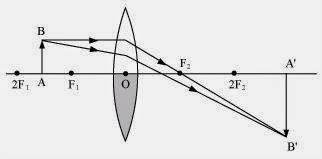When the lower half of the lens is covered
In this case, a ray of light coming from the object is refracted by the upper half of the lens. These rays meet at the other side of the lens to form the image of the given object, as shown in the above figure.

10. An object 5 cm in length is held 25 cm away from a converging lens of focal length 10 cm. Draw the ray diagram and find the position, size and the nature of the image formed.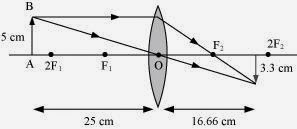11. A concave lens of focal length 15 cm forms an image 10 cm from the lens. How far is the object placed from the lens? Draw the ray diagram.

Focal length of concave lens (OF1), f = - 15 cm
Image distance, v= - 10 cm
According to the lens formula,The negative value of u indicates that the object is placed 30 cm in front of the lens. This is shown in the following ray diagram.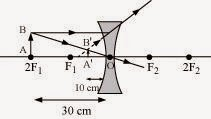12. An object is placed at a distance of 10 cm from a convex mirror of focal length 15 cm. Find the position and nature of the image.

Focal length of convex mirror, f= +15 cm
Object distance, u= - 10 cm
According to the mirror formula,The image is located at a distance 6 cm from the mirror on the other side of the mirror.
The positive and value less than 1 of magnification indicates that the image formed is virtual and erect and diminished.

13. The magnification produced by a plane mirror is +1. What does this mean?

The positive sign means image formed by a plane mirror is virtual and erect. Since the magnification is 1 it means that the size of the image is equal to the size of the object.

14. An object 5 cm is placed at a distance of 20 cm in front of a convex mirror of radius of curvature 30 cm. Find the position, nature and size of the image.

Object distance, u= - 20 cm
Object height, h= 5 cm
Radius of curvature, R= 30 cm
Radius of curvature = 2 × Focal length
R= 2f
f= 15 cm
According to the mirror formula,The positive value of image height indicates that the image formed is erect.
Therefore, the image formed is virtual, erect, and smaller in size.

15. An object of size 7.0 cm is placed at 27 cm in front of a concave mirror of focal length 18 cm. At what distance from the mirror should a screen be placed, so that a sharp focused image can be obtained? Find the size and the nature of the image.

Object distance, u= - 27 cm
Object height, h= 7 cm
Focal length, f = - 18 cm
According to the mirror formula,The negative value of image height indicates that the image formed is inverted.

16. Find the focal length of a lens of power -2.0 D. What type of lens is this?

Power of lens, P= 1/
P= -2D
f= -1/2 = -0.5 m
A concave lens has a negative focal length. Hence, it is a concave lens.

17. A doctor has prescribed a corrective lens of power +1.5 D. Find the focal length of the lens. Is the prescribed lens diverging or converging?

Power of lens, P= 1/f
P= 1.5D
f= 1/1.5 = 10/15 = 0.66 m
A convex lens has a positive focal length. Hence, it is a convex lens or a converging lens.

Go Back To NCERT Solutions for Class 10 Science

## Chapter 10 Light Reflection and Refraction Class 10 NCERT Solutions

NCERT Solutions for Class 10 Science will become your comprehensive guide in easy learning and evaluating yourself. Students should also refer previous year questions and practise test papers and worksheets to assess their key areas. Chapter 10 of NCERT Solutions Class 10 Science consists of 17 exercise questions and 14 in-text questions.

Topics in the Chapter

• Reflection of Light
• Spherical Mirror
→ Image Formation by Spherical Mirrors
→ Representation of Images Formed by Spherical Mirrors Using Ray Diagrams
→ Mirror Formula and Magnification
• Refraction of Light
→ Refraction through a Rectangular Glass Slab
→ The Refractive Index
→ Refraction by Spherical Lenses
→ Image Formation by Lenses
→ Image Formation in Lenses Using Ray Diagrams
→ Sign Convention for Spherical Lenses
→ Lens Formula and Magnification
→ Power of a Lens

### NCERT Solutions for Class 10 Science Chapters:

FAQ on Chapter 10 Light Reflection and Refraction

#### Why do we prefer a convex mirror as a rear-view mirror in vehicles?

We prefer a convex mirror as a rear-view mirror in vehicles because a convex mirror gives an erect and diminished image. As a result, convex mirror help the driver to have much wider field view.

#### What is meant by power of a lens?

The power of a lens is defined as the reciprocal of its focal length (I) expressed in meters. SI unit of power is diopter. One diopter is defined as the power of a lens whose focal length is 1 meter.

#### What is absolute refractive index?

When first medium is taken as vacuum, the refractive index of second medium is called as absolute refractive index.

#### When a convex lens will act as magnifying Glass?

When the object is kept on focal length of the convex lens, it is called magnifying glass.

#### Which mirror give an erect and enlarged image of an object?

Concave mirror give an erect and enlarged image of an object.